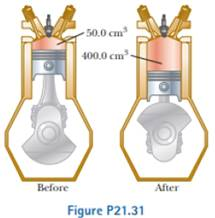# During the power stroke in a four-stroke automobile engine, the piston is forced down as the mixture of combustion products and air undergoes an adiabatic expansion. Assume (1) the engine is running at 2 500 cycles/min; (2) the gauge pressure immediately before the expansion is 20.0 atm; (3) the volumes of the mixture immediately before and after the expansion are 50.0 cm 3 and 400 cm 3 , respectively (Fig. P21.31); (4) the time interval for the expansion is one-fourth that of the total cycle; and (5) the mixture behaves like an ideal gas with specific heat ratio 1.40. Find the average power generated during the power stroke.### Physics for Scientists and Enginee...

9th Edition
Raymond A. Serway + 1 other
Publisher: Cengage Learning
ISBN: 9781305116399

#### Solutions

Chapter
Section### Physics for Scientists and Enginee...

9th Edition
Raymond A. Serway + 1 other
Publisher: Cengage Learning
ISBN: 9781305116399
Chapter 21, Problem 21.31P
Textbook Problem
5 views

## During the power stroke in a four-stroke automobile engine, the piston is forced down as the mixture of combustion products and air undergoes an adiabatic expansion. Assume (1) the engine is running at 2 500 cycles/min; (2) the gauge pressure immediately before the expansion is 20.0 atm; (3) the volumes of the mixture immediately before and after the expansion are 50.0 cm3 and 400 cm3, respectively (Fig. P21.31); (4) the time interval for the expansion is one-fourth that of the total cycle; and (5) the mixture behaves like an ideal gas with specific heat ratio 1.40. Find the average power generated during the power stroke.To determine

The average power generated during the power stroke.

### Explanation of Solution

Given info: The no of cycles is 2500cycles/min , the pressure before expansion is 20atm , the initial volume is 50cm3 and the final volume is 400cm3 , the expansion time is 14 of no of cycles and the specific heat ratio is 1.40 .

Write the expression for adiabatic process.

P1V1γ=P2V2γ

Here,

P1 is the initial pressure.

P2 is the final pressure.

V1 is the initial volume.

V2 is the final volume.

Rearrange the above expression to get P2 .

P2=P1V1γV2γ

The value of adiabatic index for a diatomic gas is 1.4 .

Substitute 20atm for P1 , 400cm3 for V2 and 50cm3 for V1 .

P2=(20atm)(50cm3400cm3)1.4=1.088atm×1.013×105Pa1atm=1.10×105Pa1×105Pa

Thus the final pressure is 1×105Pa .

Write the expression for energy transferred by heat.

Q=nCVΔT=n(52R)ΔT=52(nRΔT)=52(P2V2P1V1)

Here,

n is the no of moles

### Still sussing out bartleby?

Check out a sample textbook solution.

See a sample solution

#### The Solution to Your Study Problems

Bartleby provides explanations to thousands of textbook problems written by our experts, many with advanced degrees!

Get Started

Find more solutions based on key concepts
What is the ultimate function of the whole system?

Chemistry for Engineering Students

Find an article in a newspaper or magazine or on the Internet that makes claims about saturated fat, particular...

Nutrition: Concepts and Controversies - Standalone book (MindTap Course List)

What physical conditions cause jaundice?

General, Organic, and Biological Chemistry

Where did the Earths heavy elements come from?

Oceanography: An Invitation To Marine Science, Loose-leaf Versin

How do you recognize the formula of an acid?

Introductory Chemistry: An Active Learning Approach